Study Guide

# The Basics of Circles

## The Basics of Circles

Mathematicians like to define things before they start talking about them. This is a good idea in other areas of life too. Ever brought a cake pan to a baseball game because you heard there would be a batter?

Given a point O and a distance r, the circle with center O and radius r is the set of all points in a plane that are exactly r units away from O. Easy enough, right?

• ### Center and Radius

According to our definition, two elements define a circle: the center (a point) and the radius (a distance). If you know both the center and the radius of a circle, then you can draw the circle. Conversely, to draw a circle, you need to know both its center and its radius. It's all about give and take, isn't it?

Think about it like this: Since every circle is the same shape (uh…round), the only things that distinguish two circles are where they are and how big they are. The center tells you where the circle is. The radius tells you how big it is.

Here we have a circle with center O (written ⊙O). Point A is on the circle, making the distance OA the radius of the circle.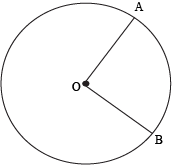Unfortunately, there's a little ambiguity in the term "radius." We use it to refer to a line segment with endpoints at the center of the circle and a point on the circle as well as the length of such a segment. In the figure above, OA and OB are radii of ⊙O.

Since all radii of the circle have the same length (the radius of the circle), we can prove that any two radii of the same circle are congruent.

For instance, given that points A and B are on ⊙O, we can prove that OA and OB are congruent using the definition of a circle. The distances OA and OB are both equal to the radius of the circle because all points on the circle are equidistant from O. In other words, OA = OB. By definition of congruence of line segments, segments OA and OB are congruent.

We might call this the "wheel theorem," since it's what makes wheels work. Seriously, it's "wheely" important.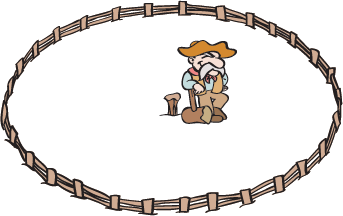Back in the pioneer days, a homesteading family (call them the Smiths) might have driven their wagon out into the middle of a flat, wide-open plain of untamed wilderness, hammered a stake into the ground, and claimed "all land within ten miles of the stake" as their property. What shape would the Smith family's property be?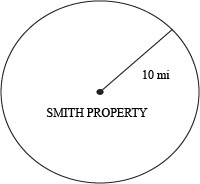Looks like a circle, right? It even has a center (the stake) and a radius (10 miles.) According to our definition, though, a circle is only the set of points exactly a certain distance away from the center, whereas the Smiths' property includes all points within that distance. A fence enclosing the property might be considered a circle, but the Smiths own more than the fence. Otherwise there's not much point in building the fence at all, is there?

So, while the boundary of the property is indeed a circle (with radius 10 miles and center at the stake), the entire property is technically the inside of that circle—a region we call a disk. If it helps, you can think of CDs and not pioneers.

The Smiths' fence divides the world into three regions: the set of points inside the fence, the set of points outside the fence, and the set of points on the fence. Similarly, any circle divides the plane into three regions.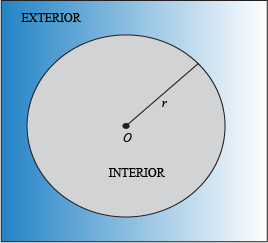Given ⊙O with radius r and a point P in the same plane as ⊙O:
P is in the interior of ⊙O if OP < r
• P
is in the exterior of ⊙O if OP > r

Finally, by the definition of a circle, we already know that P is on ⊙O if OP = r.

### Sample Problem

Suppose that circle ⊙O has a radius of 3 inches. If the distance OP is 3 inches, is P on the exterior, interior, or on ⊙O?

We can start by comparing the distance from P to O to the radius of ⊙O. In this case, OP = 3 inches, and the radius r of ⊙O is 3 inches. Since 3 = 3, we know that OP = r. By definition that means P is on ⊙O.

With a circle, we can organize and tame any wide-open plane, just as the Smiths corralled the wilderness with their stake and circular fence. And it's no coincidence that "pioneer" contains the word "pi."

• ### Central Angles

Among the most important applications of the circle to human progress has been circle-based (disk-shaped) food items. This is a family of foods that includes, among other things, cookies, bagels, doughnuts, pies, and pizzas. (Some consider pizzas to be a subset of pies, but pepperoni pie doesn't sound nearly as delicious as pepperoni pizza.)

Critical to the development of circle-based foods was the concept of the central angle. Given ⊙O, an angle is a central angle of ⊙O if its vertex is at O. Simple as that.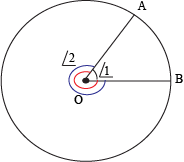Here, ∠1 is a central angle of ⊙O. So is ∠2. Every central angle has a buddy. The measure of a central angle and its buddy angle add up to 360°, the number of degrees in a full circle. In other words, buddy angles complete each other. Aww.

With the discovery of the central angle, people could easily share circle-based foods as they saw fit. If you wanted to divide a pizza among five people, you could cut slices based on central angles of 360° ÷ 5 = 72°.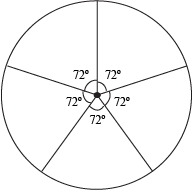### Sample Problem

It's your birthday and you'll cry if you want to. There's no reason to cry though, since you got a massive chocolatey birthday cake. If there are 20 people total at your party, at what central angle should you cut the cake?

Regardless of how big the cake is, it has a central angle of 360° because it's a circle. If there are 20 people, we should cut everyone a slice that is 360° ÷ 20 = 18° in measure. Time to bust out the protractor.

### Sample Problem

All circles are similar. True or false?

It's been a while since we talked about similarity, so here's a quick refresher: similarity exists when two figures are the same shape (all their angles are equal), but not the same size. This also means they can be carried onto each other using similarity transformations (translation, reflection, rotation, and dilation).

Circles have 360° total. That won't change ever, so we took care of the angle requirement (as well as the rotation and reflection requirements). If a single point and a length defines a circle, we can always translate the point to a different location and dilate the length so it matches another.

In other words, all circles are similar to each other because any similarity transformation can move one onto the other. In fact, only dilation and translation are needed, so we leave reflection and rotation at home.